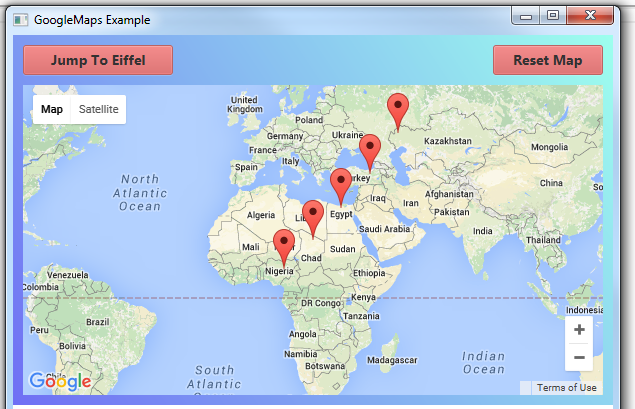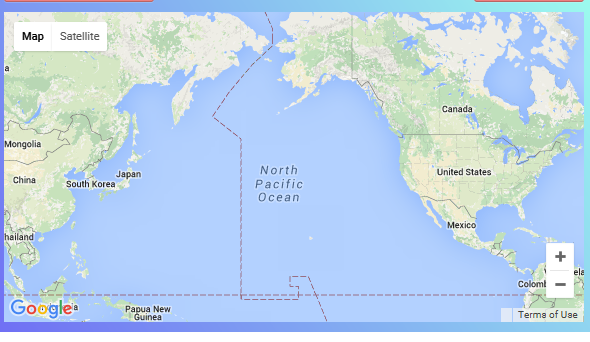# B4J Question X,Y of Pane for Jgooglemap marker

Discussion in 'B4J Questions' started by Roger Daley, Dec 27, 2015.

1. Hi All,

I am looking for away to relate the LatLng of a JGoogleMap to the X,Y of the map Pane.
It could be the X,Y of a marker or the LatLng of the corners of the displayed map.
I can do this in B4A using GoogleMap Extras but not available in B4J.

Any clues gratefully received.

Regards Roger

sor theara likes this.
2. Hi Erel,Forgot about that one, must be the Christmas fog.
Unfortunately the output appears unrelated to the screen position of the marker. No matter where the marker is located on the screen it gives the same result.

My test code is below, if you can spot a solution it would be much appreciated.

Regards Roger

Code:
`Sub btnAutoZoom_Action    Private BTSX, BTSY As Double    Private BLL As LatLng    BLL.Initialize(BTSLat,BTSLng)    Log(BTSLat)    Log(BTSLng)    Dim xy() As Double = LatLonToXY(BLL)    BTSX = xy(0)    BTSY = xy(1)    Log(BTSX)    Log(BTSY)End SubPublic Sub LatLonToXY(ll As LatLng) As Double()   Dim jo As JavaObject = gmap   jo = jo.GetFieldJO("map").RunMethod("getProjection", Null)   If jo = Null Then Return Array As Double(-1, -1)   jo = jo.RunMethod("fromLatLngToPoint", Array(ll))   Return Array As Double(jo.RunMethod("getX", Null), jo.RunMethod("getY", Null))End Sub`

Last edited: Dec 28, 2015
3. You can try the code posted here: http://krasimirtsonev.com/blog/arti...t-latlng-object-to-actual-pixels-point-object

Code:
`Dim mapJO As JavaObject = gmapmapJO = mapJO.GetFieldJO("map")Dim projection As JavaObject = mapJO.RunMethod("getProjection", Null)Dim bounds As JavaObject = mapJO.RunMethod("getBounds", Null)Dim ne As LatLng = bounds.RunMethod("getNorthEast", Null)`

4. Sorry Erel,

I stare at this code, I stare at the code on the link, I stare at the first code and I don't get started. I flounder to frame a question.
What do I do with the Jave script on the link?
Why doesn't the first code work?
Does the first code use the Java script, "fromLatLngToPoint" ?

Regards Roger

5. You can use this code:
Code:
`Public Sub LatLonToXY(ll As LatLng) As Double()   Dim mapJO As JavaObject = gmap   mapJO = mapJO.GetFieldJO("map")   Dim projection As JavaObject = mapJO.RunMethod("getProjection", Null)   Dim bounds As JavaObject = mapJO.RunMethod("getBounds", Null)   Dim ne As LatLng = bounds.RunMethod("getNorthEast", Null)   Dim sw As LatLng = bounds.RunMethod("getSouthWest", Null)   Dim topRight As JavaObject = projection.RunMethod("fromLatLngToPoint", Array(ne))   Dim bottomLeft  As JavaObject = projection.RunMethod("fromLatLngToPoint", Array(sw))   'PrintPoint(topRight, "TopRight")   'PrintPoint(bottomLeft, "BottomLeft")   Dim scale As Double = Power(2, gmap.CameraPosition.Zoom)   Dim worldPoint As JavaObject = projection.RunMethod("fromLatLngToPoint", Array(ll))   'PrintPoint(worldPoint, "WorldPoint")   Return Array As Double((worldPoint.RunMethod("getX", Null) - bottomLeft.RunMethod("getX", Null)) * scale, _     (worldPoint.RunMethod("getY", Null) - topRight.RunMethod("getY", Null)) * scale)End SubSub PrintPoint(p As JavaObject, s As String) 'ignore   Log(\$"\${s}: \$1.2{p.RunMethod("getX", Null)} x \$1.2{p.RunMethod("getY", Null)}"\$)End Sub`
It will return the correct X results only if the visible map is fully contained in the main zone.

Play with it with this code:
Code:
`Sub gmap_Click (Point As LatLng)   Dim xy() As Double = LatLonToXY(Point)   Log(\$"x = \$1.2{xy(0)}, y = \$1.2{xy(1))}"\$End Sub`
You will see what I mean.

jmon and Roger Daley like this.

6. Erel,

Thanks for the code, it works welll.
Unfortunately, playing with the code still leaves me uncertain as to what you refer when you say, "the visible map is fully contained in the main zone". I am guessing that you mean that if I zoom out far enough to display multiple views of the world map/marker, "X" is then in doubt.

I will use the code as a training example so I can hopefully ask more intelligent questions in the future.

Regards Roger

7. More or less.

Good:Not good:Roger Daley likes this.
8. Erel,

Now I see what you mean, should not be a problem in my project but it is strange. I will think about it.

Regards Roger.
London could be a problem.

Last edited: Dec 28, 2015
9. Hi All,

Belatedly this is the result of this thread.
The problem to which Erel refers occurs when the map contains 180degrees longtitude. ie the longtitude suddenly changes from +179.99999 to -179.99999, the projection method doesn't handle this. The code below appears to address this. In playing with Erels code I found that I didn't need X&Y so I haven't really stress tested the sub.

Regards
Roger

Code:
`Public Sub LatLonToXY(ll As LatLng) As Double()    Dim mapJO As JavaObject = gmap    mapJO = mapJO.GetFieldJO("map")    Dim projection As JavaObject = mapJO.RunMethod("getProjection", Null)    Dim bounds As JavaObject = mapJO.RunMethod("getBounds", Null)    Dim ne As LatLng = bounds.RunMethod("getNorthEast", Null)    Dim sw As LatLng = bounds.RunMethod("getSouthWest", Null)    Dim topRight As JavaObject = projection.RunMethod("fromLatLngToPoint", Array(ne))    Dim bottomLeft  As JavaObject = projection.RunMethod("fromLatLngToPoint", Array(sw))    Dim scale As Double = Power(2, gmap.CameraPosition.Zoom)    Dim worldPoint As JavaObject = projection.RunMethod("fromLatLngToPoint", Array(ll))       Dim PointX, PointY As Double    Dim EastB As Double = ne.Longitude    Dim WestB As Double = sw.Longitude    Dim MarkerLng As Double = ll.Longitude    Dim ScreenDegrees As Double    Dim DegPerPix As Double' Get X&Y by getProjection    PointX = (worldPoint.RunMethod("getX", Null) - bottomLeft.RunMethod("getX", Null)) * scale    PointY = (worldPoint.RunMethod("getY", Null) - topRight.RunMethod("getY", Null)) * scale   ' Get X 180Long when is included in map. 'Screen width is measured in both pixels and degrees, MarkerLng is known in degrees, calculated in pixels = X.    If WestB > EastB Then        ScreenDegrees = (180-WestB) + (180+EastB)        DegPerPix = ScreenDegrees/Pane1.Width        If MarkerLng > 0 Then PointX = (MarkerLng - WestB) / DegPerPix        If MarkerLng < 0 Then PointX = ((180+MarkerLng) + (180-WestB))/DegPerPix    End If    Return Array As Double(PointX, PointY)End Sub`

Erel and eurojam like this.